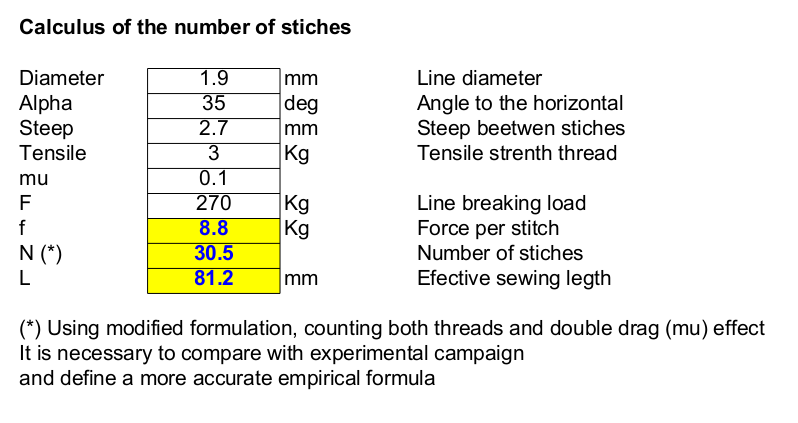LINE SEWING. THEORETICAL AND PRACTICAL ASPECTS

1. Theoretical aspectsFig 1. Loop tensile diagramFig 2. Loop equilibrium

The above formula needs to be improved as follows:
1) Consider the resistance of the thread is twice, due to the upper and lower thread.
2) The zigzag stitch consists of two types:
Traction stitches (drawn in red) work when the loop tend to slide.
Complementary stitches only work adding friction force.
Consequently. The formula can be improved, according to the following expression:

N=F / (4·T·(((sin (alpha) ) + (2·mu·cos (alpha)))Fig 3. Example of calculusFig 4. Real examples in 1.2 mm and 1.9 mm lines

2. Practical aspectsFig 5. Example 1 and 1.8 mmFig 6. Examples 1.2 mmFig 7. How to measure and cut loopFig 8. Special footFig 9. Couper et brûler truc